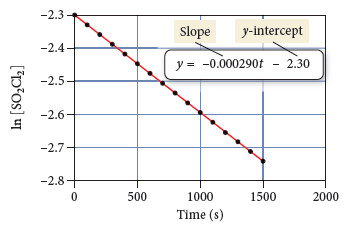⚠️Our tutors found the solution shown to be helpful for the problem you're searching for. We don't have the exact solution yet.

# Solution: Consider the equation for the decomposition of SO2Cl2: SO2Cl2(g) → SO2(g) + Cl2(g)The graph of ln[SO2Cl2] versus time isUse the graph and the best fitting line to predict the concentration of SO2Cl2 at 1800 s.

###### Problem

Consider the equation for the decomposition of SO2Cl2SO2Cl2(g) → SO2(g) + Cl2(g)

The graph of ln[SO2Cl2] versus time isUse the graph and the best fitting line to predict the concentration of SO2Cl2 at 1800 s.